# VIII. Diffraction and Microscopy

One classical method of studying the structure of matter in fine detail is to shine a beam ofradiation on a sample and observe the outgoing radiation. Examples of this approach include thevisible light and electron microscopes and the X-ray determination of the atomic coordinatesof crystallized protein molecules. We will consider all of these examples.

## A. Wave Scattering By a Point Obstacle

The fineness of detail that can be studied by a given method (the "resolution") depends on thewavelength of the radiation used (the shorter the finer), the maximum angle of scattering thatis detected (the wider the finer), and the sensitivity of the sample to damage by the type ofradiation used (the less sensitive the more information can be extracted before the structurehas changed significantly). This topic "brings you face to face with wave-particle duality"; wave interference is observed by counting x-ray photons! For historical reasons, and forconvenience in parts of the mathematical analysis, the "scattering angle" or "deflectionangle" is called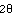; see Fig. 1.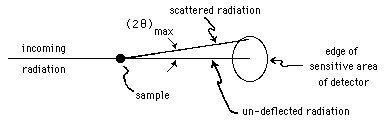Figure 1: Basic Scattering Experiment

A beam of particles all traveling in the same direction are described mathematically asplane-waves. Particles headed in toward a common focus are described as ingoing sphericalwaves, and particles diverging from a common center are outgoing spherical waves. Thus wecan see that the effect of a point obstacle in scattering a beam of particles may be described asdiminishing the intensity of the plane waves and creating outward-going spherical wavescentered on the obstacle. The spherical waves will not typically be equally intense in alldirections, but will instead usually be more intense at small scattering angles.

Such scattering must be divided into two classes, based on the relationship between the incidentplane waves and the outgoing spherical waves. The various physical mechanismsresponsible, depending on the nature of the radiation and the obstacle, may produce eitherclass, or even both classes at once. The first class is "coherent scattering," in which thefrequency and wavelength of the outgoing spherical waves are the same as the incident waves,and in which there is some fixed phase relationship, at the obstacle, between the two sets ofwaves. This is a case of "elastic scattering."

The second class of events is everything else, known collectively as "incoherent scattering." If the wavelength is shifted, the particle's energy and momentum will have been changed, sothis class does include any "inelastic scattering." Compton scattering of photons by atomicelectrons is an example of such a process. Because the incident waves cannot provide a link tocoordinate the outgoing waves from the various atoms of a crystal, these incoherent processesare not responsible for any systematic reinforcement or cancellation of the waves scatteredfrom a crystal. In X-ray diffraction from a protein crystal, for example, four-fifths of thephotons pass through undeflected and almost one-fifth are absorbed or are scatteredincoherently. Typically only 1% will be coherently scattered to form the diffraction pattern.

## B. Crystals

A crystal may be loosely defined as a piece of solid matter in which the atoms are regularlyarrayed in space. An example is aluminum, in which the arrangement is described as"face-centered cubic" or "FCC," see Fig. 2.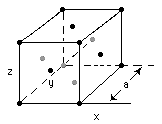Figure 2: FCC Lattice

The FCC lattice has atoms at the corners of the cubes and at the centers of the faces. This pattern is repeated throughout the crystal; in Fig. 2 the atoms on the three "far" faces and the "back" corner are shown as gray, while the atoms on the three "near" faces and the other corners are shown as black, as an aid to visualizing their three-dimensional locations, although of course all the atoms in an Aluminum crystal are identical. Each corner atom is shared by eight cubes, and eachface-center atom is shared by two cubes. (The FCC crystal can also be viewed as made up ofrhombohedral cells, with the face-center atoms at the corners, and the other two corners theatoms with (x,y,z) = (0,0,0) and = (a,a,a); see Kittel,1966, Chapter 1.)

There are many ways to place planes within the crystal so that atoms throughout the crystalwill fall in the plane. For example, in an FCC structure, the planes of atoms with z = 0, x = a/2,z = a, etc.. The planes z = 0 and z = a are equivalent to each other, but not to the plane z = a/2. Many of the sets of equivalent planes (all planes in such a set being parallel) will haveseparations between adjacent planes other than a, especially those at odd angles. A method forsystematically naming the sets of planes will be discussed below.

## C. The Interference of Scattered Waves

The physical process by which the radiation of an incoming beam is scattered by a crystal will depend on the nature of the radiation (electrons, neutrons, X-rays, etc.), but the mathematical descriptions are remarkably similar: they are all based on the quantum mechanical wave nature of the radiations. Where the following discussion seems specific to X-ray photon scattering, it can be generalized to cover other radiations. As discussed above, each atom scatters waves to become spherical outgoing wavefronts, and these wavefronts from each of the atoms superimpose in constructive or destructive interference, depending on their relative phase and amplitude, as determined by the wavelength, the locations of the atoms, and the direction of the outgoing radiation relative to the incoming radiation and the orientation of the crystal.

Systematic interference, in which the waves in certain directions are continually reinforced, will occur only for the so-called "coherent scattering" processes, for example Thomson scattering of photons. Radiation emitted from the crystal due to fluorescence caused by photon bombardment would be omni-directional, since each excited atom would radiate independently of the others. Coherently scattered radiation does not come from each atom independently; instead, the waves radiated from each atom are coordinated by their common origin from the X-ray source and the phase-sensitive scattering processes. This produces scattered radiation that is weak or zero in intensity in most directions, and quite strong in certain particular directions.

We will examine two equivalent mathematical forms for the analysis of such crystaldiffraction. The Bragg analysis is more intuitive in trying to grasp the essence of what ishappening, but the Fourier analysis is the one used for calculation of complex structures.

### The Bragg Analysis

Sir Laurence Bragg's insight was that the problem of predicting the diffraction pattern can bebroken down into three parts: first, in what direction will the radiation go that has been reflected by a singleplane of atoms? Second, will the partial reflections from all of the other equivalent planes(parallel to that plane) reinforce or cancel each other? Third, how strong will the reflection fromeach plane be? The first question is simply answered: the planes reflect just like ordinarymirrors, as in Fig. 3(a). This will be true if all the atoms of the plane give the same phase shiftfor their outgoing spherical waves.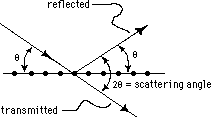Figure 3(a): Partial reflection and transmissionby a plane of atoms.

Figure 3(a) also illustrates the origin of analyzing in terms of theta, half the scattering angle. It is the grazing angle, the supplement of the angle to the normal that is conventionally used in studying optical reflections.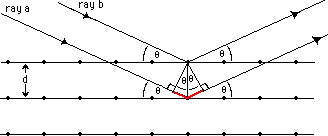Figure 3(b): Path-length difference by ray tracing for partial reflectionsfrom consecutive equivalent planes of atoms.

The answer to Bragg's second question is based on Fig. 3(b), an analysis that is very similar to the classical two-point interference problem. The waves scattered by adjacent planes will reinforce only at angles such that the total path-length difference is an integer multiple of the radiation wavelength,. The path of ray a is longer than that of ray b, by the two segments drawn as a thicker, red line in Fig. 3(b). Each of those two segments is of length dsin(). From this we get Bragg's Law for the angles of reinforcement: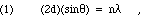where n is the integer order number and d is the separation between adjacent equivalent planes of the set.

### Fourier Analysis

For those whose mathematical background includes such things, we can say that the diffraction pattern represents the Fourier transform of the scattering power of the sample. Thus in the case of X-ray diffraction the pattern is the transform of the atomic electron density throughout the crystal. The mathematics demonstrates clearly that for a perfectly ordered crystal this will result in a diffraction pattern of isolated spots, that is, beams of reinforced scattered radiation at specific angles.

The intensity of each beam and its relative phase are determined by the structure within each unit cell of the crystal, while the directions of the beams are determined by the three dimensional regularity of the packing of the unit cells within the crystal. As will be discussed later under the heading "Structure Studies," the observable quantity is reflection intensity, whereas to calculate the electron density (by Fourier techniques for instance) requires also a knowledge of the relative phases of the waves in each reflected beam.

### Crystal Plane Nomenclature

We will return to Bragg's third question after considering notations for describing the various planes of atoms. One way to specify a plane is to give the co-ordinates of any three non-colinear atoms lying in that plane. Although logically adequate to specify the plane, this information is not in a very useful form. Alternatively, if a rectangular crystal is set with its axes parallel to the x, y, and z coordinate axes, the plane could be specified by the direction and length of the shortest vector from the origin to the plane (a vector which will of course be perpendicular to the plane). Again the information is in an awkward form. The standard method for specifying the planes is known as Miller Indices, which are computed from the intercepts of the plane with the coordinate axes.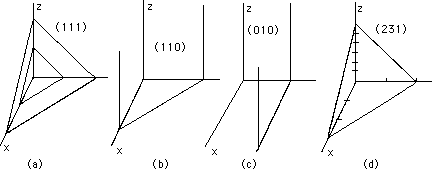Figure 4: Bragg Planes and Miller Indices

We work an example calculation of Miller indices, based on Fig. 4(e): let a be the lattice spacing in the x-direction, b in the y-direction, and c in the z-direction. (For a cubic crystal, a = b = c.) Suppose for example that the plane cuts the axes at 3a, 2b, and 6c. Write the reciprocals of the coefficients (that is, the lattice spacings divided by the intercepts), 1/3, 1/2, and 1/6, and express them using their least common denominator: 2/6, 3/6, and 1/6. The three numerators are the Miller indices for that plane (and for any parallel plane, Fig.4(a)). In general the Miller indices are written (hkl), for our example, (231). A plane parallel to a coordinate axis will have intercept at infinity, thus its index is 1/infinity = 0. Although the method of calculating the Miller indices may seem quite obscure in this context, these indices show up repeatedly in the various formal mathematical analyses of crystal diffraction of radiation. The separation between Bragg planes, d in Eq. 1, can be calculated by geometry from the Miller indices and a knowledge of the unit cell parameters a, b, and c. The (hkl) designation for a spot is identified in the Fourier analysis as the harmonic (overtone) numbers in each dimension.

The crystal plane-spacing distance, d, can be deduced from macroscopic measurements, as shown here. The mass of a molecule is M/N, which will be in g/molecule if M is the molecular formula weight and N is Avogadro's number. The number of molecules per unit volume is/[M/N], with units of molecules/cubic meter ifis the density in g/cubic meter. For diatomic molecules, such as NaCl and LiF, the number of atoms per unit volume is 2N/M. The distance between adjacent atoms, d, in crystals of diatomic molecules is therefore given by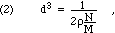The value of Avogadro's number is here used to calculate d. If, however, the wavelength of the radiation is obtained by using a man-made grating, as can now be done, then one may reverse the sequence of Bragg's argument to provide an accurate physical evaluation of Avogadro's number.

### Polycrystalline Diffraction

A polycrystalline sample consists of many "fragments," each one a good crystal that is large compared to the wavelength of the incident radiation. We can therefore treat the interaction between each fragment and the beam separately. For most sets of planes (hkl) and most orders, n, the orientation to the beam for any one fragment will not produce a reinforced interference. However, in a macroscopic sample there are so many possibilities that there will likely be many crystal fragments correctly aligned for each combination of (hkl) and n. Rotation of the crystal fragment about the incoming beam line leaves the Bragg condition (Eq. 1) satisfied, but moves the diffracted beam around an arc, defining a cone. Individual fragments each give a single spot for a particular (hkl) and n combination, but the collection of randomly oriented fragments will give a series of coaxial cones of scattered radiation, all with vertex at the sample, producing concentric circles at the intersection with a plane detector aligned perpendicular to the main beam. See Fig. 5; each circle corresponds to a particular combination of (hkl) and n (or perhaps, by coincidence, to two or three combinations).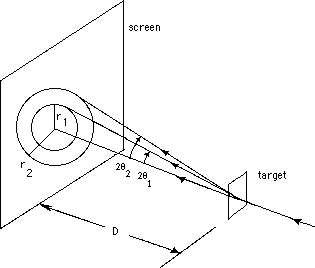Figure 5: Polycrystalline Diffraction

## D. Wave Radiation Sources

In order to perform diffraction structure studies it is best to have a source of "monochromatic radiation" (all quanta having the same wavelength). In the case of electrons, this can be done adequately for most cases by creating the beam with a conventional electron gun (such as is used in a CRT), and accelerating them with an applied potential. The variation in wavelength is then caused by variations in energy from failure to regulate the accelerating potential and from thermal fluctuations of the electrons' initial velocities, especially if they were "boiled off" of a hot cathode.

For neutrons and X-rays the usual approach is to create a beam that is not purely one wavelength, as discussed in chapter III, and then to run it through a "monochromator" that rejects all but one wavelength (or a narrow range of wavelengths, more likely). Frequently this will be done by diffraction from a simple, sturdy crystal (NaCl salt, graphite, Si, etc.). In such cases there will be a trade-off: the wider the acceptance angle of the outgoing beam from the monochromator, the stronger the usable beam but also the wider the range of wavelengths present.

For the purposes of X-ray diffraction structure studies, it is often adequate to select an electron beam kinetic energy that enhances the proportion of photons in one of the characteristic lines, and then to pass the beam through a foil of an element chosen to selectively absorb whichever other characteristic line is most likely to create problems. For difficult cases, of course, one can use a crystal positioned to pick out the desired characteristic line by Bragg diffraction.

### Matter Waves

The wavelike nature of matter was predicted by Louis de Broglie and demonstrated by Davisson and Germer. The wavelength associated with a particle (electron, neutron, etc.) in motion can be calculated from the following. The (b) forms of Eqs. 3 through 6 are relativistically correct; the (a) forms are approximations that are valid if v << c.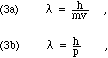where h is Planck's constant, p is the particle's momentum, m is its mass, and v is its velocity. If the particles are electrons, the potential used to accelerate them, V, can be used to rewrite Eq. 3, as follows: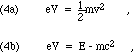where e is the electron's charge, m is its mass, c is the speed of light, and E is the particle's total energy (including rest energy). Recall, from the theory of relativity (see, for example, Okun, 1989), that the invariant magnitude of the momentum-energy 4-vector gives us the relationship that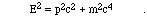Eliminating v, the electron's velocity, between Eqs. 3a and 4a, and eliminating p, the electron's momentum, between Eqs. 3b and 4b, yields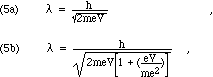Substituting the presently accepted values for e, m, and h gives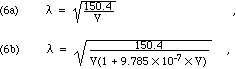where V is in Volts andis in Angstroms. Observe that the relativistic correction towill be less than 10 percent unless V exceeds 240,000 Volts.

There are two conventional techniques for producing a beam of electrons of well-defined energy. The simpler is the "electron gun," such as is found in cathode-ray tubes for oscilloscopes or television receivers. The kinetic energy of electrons accelerated in this way rarely exceeds 200 keV. For higher energies, modified forms of the "atom smashing" particle accelerators are used. A LINAC, so called because it accelerates the electrons along a line instead of the circular or spiral paths of a synchrotron or cyclotron, can produce electrons of more than 3 MeV, and can be used directly or as part of the internal structure of an x-ray generating machine (see Figs. III-1 and VII-1, and their accompanying discussion).

The two major neutron sources for diffraction studies are nuclear reactors, which generate copious quantities of neutrons, and several radioactive nuclides. These latter neutron sources can be of relatively weak intensity for instructional or research purposes. In most of these sources the majority of the neutrons are emitted following the interaction of an initial decay's alpha particle with a lighter nucleus, such as Be (see chapter III, part C, and also Cember, 1969, p. 136).

### Synchrotron Radiation and Monochromators

A synchrotron is a particular type of circular accelerator used to boost electrons or other charged particles to very high kinetic energy. The electrons are forced by magnets to follow a curved path and synchronized electric fields are applied to increase their speed on each revolution. Since their path is curved, the velocity vector is constantly rotating and hence their acceleration is not zero. The electrons therefore radiate a continuous spectrum of photons, in exact analogy to bremsstrahlung.

Because of its continuous spectral content, synchrotron radiation can be used for diffraction study only if some way can be found to eliminate, or at least drastically reduce, all but some particular wavelength. (In analogy to visible light, "single color," the devices used are known as monochromators.) The simplest conceptually is a diffraction (grating or crystal) type. The only radiation used for the structure study is that which has already revealed its wavelength by diffracting from a known crystal at some particular angle. The intensity of the radiation at the desired wavelength can be increased by so-called "wiggler" magnets, a set of magnets with alternating orientations along the beam path, which deflect the beam sideways in alternate directions. They are constructed with spacing along the beam such that at the particle velocity of the beam, the resulting lateral accelerations occur at the desired frequency.

As mentioned previously, sometimes it will be sufficient to just pass the initial radiation through a filter that strongly absorbs all but a small range of wavelengths. Sometimes a combination of two or three such filters will produce an acceptably unique wavelength for the output radiation.

For neutrons and protons traveling appreciably slower than the speed of light, mechanical monochromators can be made that pass only particles moving with a particular speed (hence specific momentum and hence specific wavelength). The standard type is known as a "time of flight monochromator." For charged particles, such as electrons and protons, one can select the momentum, and hence wavelength, by means of the deflection caused by a transverse magnetic or electric force. The radius of curvature may be used (a path between concentric hemispheres) or the direction of the outgoing beam (reminiscent of a CRT).

## E. Diffraction Structure Studies

To answer the third Bragg question ("How strong will the reflection from each plane be?") one considers the interference of the waves from the atoms within a single plane of the (hkl) set. This is a two-dimensional problem, and is therefore considerably more complex in general than the analysis of Fig. 3(b), which is essentially one-dimensional. The usual approach considers first the "structure factor," the spot intensity that would be expected for a very large perfect crystal and a unit beam strength, and then considers as separate factors the corrections for finite crystal size, crystal defects, and the actual strength of the incoming beam of radiation. The structure factor will depend on five things, first the coordinates of the atoms, second, the differences in scattering from the individual atoms, third, the Miller indices for the set of planes, fourth, the superposition of the scattered radiation (sine waves of differing amplitude and phase but of the same wavelength), and fifth, the fact that the observed intensity will be proportional to the square of the absolute value of the wave amplitude. Some crystals have their atoms so symmetrically arrayed in space that some of the structure factors are exactly zero. In general the structure factors will range over several orders of magnitude.

Although a moderately complicated calculation in practice, especially with the large numbers of atoms in biologically interesting compounds, computing the diffraction pattern to be expected from any arrangement of atoms is simple compared to the inverse problem of deducing the atomic coordinates from an observed diffraction pattern. The heart of the difficulty is the fifth item in the previous paragraph: the observed quantity is the intensity, which is always a positive number, the square of the amplitude of the wave. In order to deduce the atomic structure from the diffraction pattern, one must know the phases of the diffracted waves at each of the thousands of spots in the pattern. Simple structures can be solved by guessing the phases, calculating the atomic structure, calculating a predicted diffraction pattern from it, and improving the phase guesses. For more interesting structures there are elaborate experimental and analytical techniques that together permit the calculation of the phases and the structure. The difficulty being in those cases mostly one of growing useful crystals of closely related derivative compounds, so that comparisons can be made between the diffraction patterns. This technique is known as multiple isomorphous replacement (MIR); see Judson, 1979.

Karle (1989) presents a good introduction to both the MIR method and to the methods he has developed using multiple-wavelength anomalous dispersion (MAD), in which the diffraction pattern is measured using several wavelengths close to an absorption edge for one of the atomic species present in modest concentrations in the molecule under study. The MAD technique is especially valuable for macromolecules for which suitable derivative compounds cannot be synthesized in adequate amounts.

Although X-ray diffraction studies have gotten the most attention, biologically interesting specimens have been studied by diffraction of electrons and of neutrons. Among the structures studied so far by one form of diffraction or another are included the following: genetic DNA, the various RNA's, enzymes and other proteins, muscle fibers (which contain two types of polymers interdigitated and with cross-sections showing an hexagonal array pattern of packing of the fibers), and membranes (which can often be, or are naturally, formed into vesicles that can be gracefully collapsed onto each other, deflated and stacked up like pancakes). This last provides a two-dimensional regularity that permits diffraction study. The major question with such membranes is typically the orientation, location and structure of membrane-bound proteins. It is difficult to imagine active transport (for example of calcium ions across a membrane) by a protein that does not fully penetrate the membrane, yet it is known that some membrane-bound proteins project on only one side.

## F. Light Microscopy

Figure 6 shows the basic features of a conventional microscope. The lenses shown as simple thin lenses are in practice more likely to be compound lenses, constructed from several separate lens elements, either glued together or separated by air gaps. This complexity in practice is required in order to compensate for the fact that the index of refraction depends on wavelength in all available lens materials. By combining elements made from different materials, with different wavelength dependence, it is possible to construct a compound lens that exhibits a focal length that is more nearly uniform for all wavelengths of visible light. Such a lens is called "achromatic" if it achieves exactly the same focal length for two different frequencies of light, and "apochromatic" if it achieves exactly the same focal length for three different frequencies of light.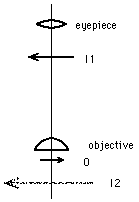Figure 6: Simplified Conventional Microscope.

The "objective" lens, so-called because it is the one closest to the object under study, is likely to be a plano-convex lens, as shown, with the plane side down, so that it can be brought quite close to the object. The object under study, O, is illuminated from below, and the light spreading from it is focussed by the objective lens to form a real image, I1, which in turn is examined by the eyepiece, used as a "magnifying glass," so that the observer sees an enlarged virtual image, I2.

### Resolution

Even if the magnification from O to I1 to I2 is large enough, it may still be impossible to see whether two closely spaced objects are in fact separated or connected. This ability to distinguish closely spaced objects is referred to as "resolution" or "resolving power." Since the issue is the brain's ability to perceive the distinction between two closely spaced separate objects and one oblong object, there are no hard and fast mathematical expressions. However, the origin of the problem is diffraction, scattering, and interference of light waves, which is subject to physical analysis, and a good rule of thumb is that the best resolvable separation (the shortest distance between two objects on the slide that the observer can be reasonably confident is not one oblong object) will be approximately as given by Eq. 7.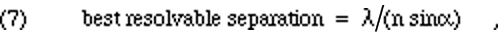whereis the wavelength of the light, n is the index of refraction of the material between the object and the first ("objective") lens, and the acceptance angle,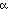, is the largest change of direction of light rays as they pass through the object (scattering angle) that still permits them to pass through all the lenses to reach the observer.

There are therefore three techniques that are used to improve the resolution of microscope observations:

1. Use light of a shorter wavelength; using blue light instead of red would give nearly a factor of two.

2. Use conically converging illumination so that the light can be scattered by twice the angle and still proceed through to the observer.

3. Fill the region between the objective lens and the object under study with a material of high index of refraction; such "oil immersion" lenses may have n as large as 1.5.

### Contrast

Even if the magnification and resolution are adequate to the task at hand, it may still be impossible to see anything useful. Biological specimens are notoriously transparent, which is easy to understand since they are largely composed of water, so that one part looks just like any other part. The variation of intensity of the light from one region to another is called "contrast." The human eye and brain are specifically evolved to detect and pay attention to contrast between adjacent regions.

Adequate contrast in the final observed image can be obtained in five ways.

1. As a result of variations in the intensity of transmitted (unscattered, unabsorbed) light. This is the conventional approach; it typically requires staining the sample, which may cause artifacts.

2. As a result of variations in the intensity of scattered light. This requires the blocking of any light which was simply transmitted directly through the sample, resulting in a dark field of view in the absence of the sample, hence the name "dark field microscope." There is less often a need for stain, hence one has more confidence about the natural existence of the observed structures.

3. As a result of variations in the interference between scattered and unscattered light. To control this you must be able to phase shift and to filter one or the other, typically the unscattered light. The need for stain is typically much reduced in phase contrast microscopy, so that even greater confidence can be placed in the observations made. Phase contrast microscopy is discussed in more detail below.

4. As a result of variations in the polarization of the light caused by passage through the sample. Such microscopes can be implemented with linear polarizers, quarter-wave plates, etc., located both before the sample and after it. If, for example, a linear polarizer is located before the sample, and another one turned perpendicular to it is located after, then no light will be transmitted, except from those parts of the sample that rotate the polarization or linearly polarize the light at some other angle.

5. As a result of variations in fluorescence or phosphorescence: the intensity is observed at wavelengths greater than those of the illumination. A sharp cut-off filter removes the illuminating wavelength light between sample and observer. Fluorescence microscopy can be used to correlate chemical activity with physical structures.

F. Zernike (1888-1966) invented the phase contrast microscope in 1935 and was awarded the Nobel Prize in 1953 for that invention. A phase contrast microscope can usually also be used for dark field microscopy, since it has arrangements that permit treating the unscattered light differently from the scattered. Figure 7 provides an example of the arrangements for a phase contrast microscope. The concave mirror, M, forms a real image of the extended light source, S, in the plane of the diaphragm, D. The diaphragm thus becomes an extended secondary source of light, with rays diverging upward from each point within the annulus.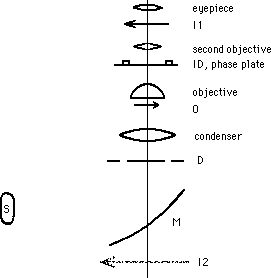Figure 7: Simplified Phase Contrast Microscope.
(After Jenkins and White, 1976.)

As this light passes upward through the condenser lens, it is refracted to create conically converging illumination of the object under study. Because the diaphragm is located at the focal distance from the condensing lens, the light diverging from a single point in the annulus will form a set of parallel rays that cross the axis on the other side of the condenser. Because the points of the annulus are about equidistant from the axis, the crossings of the axis will all occur at about the same distance from the condenser. This is where the slide stage is located. The advantage of such converging illumination is that the acceptance angle,, is twice as large as it would be with illumination arriving at the object parallel to the axis. Thus, according to Eq. 7, we can expect significantly improved resolving power.

The condenser lens and first objective lens together form a compound lens that has a shorter focal length than either alone. Hence, the diaphragm is located outside of the focal distance from that compound lens, and they therefore form a real image, ID, of the diaphragm, above the first objective, closer to it than the real image I1 of the object under study. The image ID is formed from the light that was transmitted unscattered through the sample.

The phase plate, P, is located in the plane of that real image, ID. There are several possible constructions for the phase plate. If it is simply opaque at the location of the image of the diaphragm, and transparent elsewhere, then the real image, I1, of the sample, formed near the eyepiece by the compound lens system consisting of the first and second objective lenses, will consist only of light that was scattered as it passed through the sample. This, then, is a dark field microscope.

On the other hand, the phase plate could (and typically does) have, at the location of the image of the diaphragm, a transparent annular layer whose thickness is slightly less (or more) than its thickness elsewhere. This produces a quarter wavelength phase advance (or delay) of that light compared to the light that was scattered in passing through the sample and so travels through the plane of the phase plate at other locations. If this layer also includes suitable filters, the intensity of the unscattered light can be reduced to be comparable to that of the scattered light. In that case the interference between the scattered and unscattered light at the real image of the sample, I1, may range from fully constructive to fully destructive, the maximum possible contrast.

## G. Electron Microscopy

The advantage of electron microscopy over light microscopy is simply one of resolution: the electrons used have wavelengths less than 10 picometers, compared to at least 400 nanometers for visible light. Inspection of Eq. 7 reveals immediately the advantages this bestows. Much of that advantage is offset by the very small acceptance angle,, of the electrostatic and magnetostatic "lenses" that guide the electrons along their trajectories, and by their poor "optical quality": they distort the images formed. Nonetheless, it is possible to obtain images with resolution sufficiently good to reveal individual atoms!

### Specimen Damage

The difficulties of electron microscopy derive in the most part from the fact that the electrons are of high enough kinetic energy to be ionizing radiation. Thus, the specimen will be damaged even as it is being revealed. The specimen can be protected from, and made more readily visible in the electron microscrope, by being stained properly. Typical staining techniques are akin to the "bronzing" of baby shoes: gold plating is a commonly useful technique. Such treatment of the sample forcibly raises the concern with artifacts that was already mentioned for dye staining techniques with visible light microscopes.

Hainsfeld (1987) presents a particularly elegant staining technique. He has developed a method to make small gold-conjugated antibody labels. "It permits electron microscopic visualization of single antigen-binding antibody fragments (Fab') by covalently linking an 11-atom gold cluster to a specific residue on each Fab' such that the antigenic specificity and capacity are preserved."

For biological structures, the destruction of the specimen is usually accomplished at a dose of about one millicoulomb per square centimeter, which is equal to about 0.6 electrons per square Ångstrom. Therefore, the resolution, on non-repeating features, is sure to be worse than 2 Ångstrom, and 20 Ångstrom is typical.

### Contrast

Contrast in electron micrographs can be obtained by measuring any one of several quantities. For example, the current of electrons that pass through the sample, the current of scattered electrons, the current of electrons that pass through, or scatter, with the loss of a specific amount of energy. Further improvements in contrast may result with some samples from measuring and displaying the ratios of any two of these quantities.

The trade-off between resolution and contrast is a consequence of the fact that precise measurement of currents will require prolonged exposure, or large areas. Thus the practical resolution limit may come from the area needed to get meaningful current data, or it may be limited by the destruction of the sample during the measurement period.

A transmission electron microscope illuminates the entire imaged area with electrons at once. On the other hand, a scanning electron microscope focuses the electron beam down to a very small spot on the sample, and deflects the beam back and forth in a systemmatic scan, covering the entire imaged area by dwelling briefly on an array of perhaps 1,000 by 1,000 "pixels." In order to achieve equivalent image quality, the exposure (dose) in each portion of the object must be the same; therefore, the scanning electron microscope must have a very much brighter source of electrons, or the duration of the exposure process must be increased. Since there may be a million pixels, we need to achieve six orders of magnitude increase in brightness unless the exposure time can be increased. Realistically, any increase in exposure time will surely be limited to one or two orders of magnitude, so a minimum of four orders of magnitude increase in brightness over conventional electron sources is required. The technique is field emission, as discussed in chapter I (see also, Crewe, 1971).

## H. Scanning Microscopies

Scanning microscopies are based on instruments that physically move the sample with respect to the probe, correlating the signal with the position. Typical scanning patterns are the same as with a television signal: motion along a line, retrace that motion, step in the perpendicular direction, scan again, and repeat until the region of interest has been examined, then retrace in both dimensions, back to the starting point. The probe can involve a beam of photons or of material particles; or even physical contact, as by a phonograph stylus. The latter is useful for more than just instructional experiments: if the object being examined has an appropriately resilient surface, such as the polyvinylchloride that the stylus was designed to work against, it can detect surface features as small as 50 Å. One of the critical enabling technologies that has brought scanning microscopy to the forefront of active instrumentation development is advances in the positioners that provide the motion for the scanning.

Quate (1986) presents a good introduction to another technique that can provide resolution on atomic scales: scanning tunneling electron microscopy. Horizontal resolution can be 0.1 nm, and vertical resolution can be 1 pm.

The final displayed image may be produced by analog means, in which the brightness of the light exposing the corresponding part of the film is proportional to the measured current, or the current value may be digitized for computer processing before the final display is created.

Another scanning microscopy is discussed by Kino and Corle (1989), in which the probe is light of visible wavelengths, but in which the imaging is not done by conventional optical focussing, and so is not limited to dimensions based on the wavelength, as one would otherwise expect, based on Eq. 7.

Previous   Next

Dick Piccard revised this file (https://people.ohio.edu/piccard/radnotes/diffract.html) on March 20, 2017.

Please E-mail comments or suggestions to piccard@ohio.edu.

Copyright © 2017 Richard Dickson Piccard. All rights reserved.